# Using precision to plan experiments (SPSS)

SPSS Version 28 has some new nice power analysis facilities. Here is some example code to test the difference in proportions, with sample sizes equal between the two groups, and the groups have an underlying proportion of 0.1 and 0.2.

``````POWER PROPORTIONS INDEPENDENT
/PARAMETERS TEST=NONDIRECTIONAL SIGNIFICANCE=0.05 POWER=0.6 0.7 0.8 0.9 NRATIO=1
PROPORTIONS=0.1 0.2 METHOD=CHISQ ESTIMATE=NORMAL CONTINUITY=TRUE POOLED=TRUE
/PLOT TOTAL_N.``````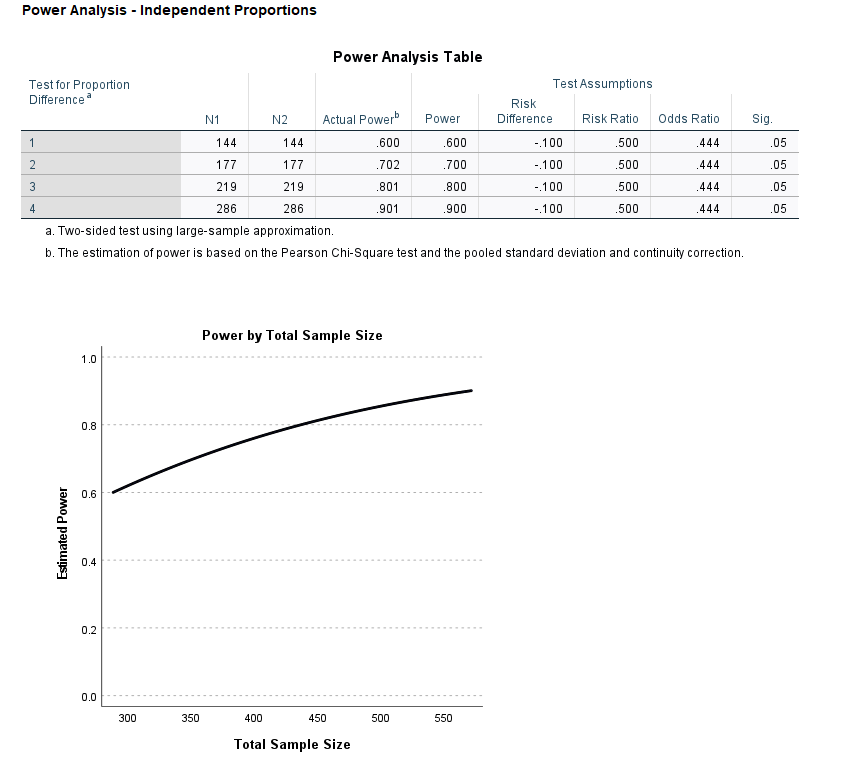So this tells you the sample size to get a statistically significant difference (at the 0.05 level) between two groups for a proportion difference test (here just a chi square test). And you can specify the solution for different power estimates (here a grid of 0.6 to 0.9 by 0.1), as well as get a nice plot.

So this is nice for academics planning factorial experiments, but I often don’t personally plan experiments this way. I typically get sample size estimates via thinking about precision of my estimates. In particular I think to myself, I want subgroup X to have a confidence interval width of no more than 5%. Even if you want to extend this to testing the differences between groups this is OK (but will be conservative), because even if two confidence intervals overlap the two groups can still be statistically different (see this Geoff Cumming reference).

So for example a friend the other day was asking about how to sample cases for an audit, and they wanted to do subgroup analysis in errors between different racial categories. But it was paper records, so they needed to just get an estimate of the total records to sample upfront (can’t really control the subgroup sizes). I suggested to estimate the precision they wanted in the smallest subgroup of interest for the errors, and base the total sample size of the paper audit on that. This is much easier to plan than worrying about a true power analysis, in which you also need to specify the expected differences in the groups.

So here is a macro I made in SPSS to generate precision estimates for binomial proportions (Clopper Pearson exact intervals). See my prior blog post for different ways to generate these intervals.

``````* Single proportion, precision.
DEFINE !PrecisionProp (Prop = !TOKENS(1)
/MinN = !TOKENS(1)
/MaxN = !TOKENS(1)
/Steps = !DEFAULT(100) !TOKENS(1)
/DName = !DEFAULT("Prec") !TOKENS(1) )
INPUT PROGRAM.
COMPUTE #Lmi = LN(!MinN).
COMPUTE #Step = (LN(!MaxN) - #Lmi)/!Steps.
LOOP #i = 1 TO (!Steps + 1).
COMPUTE N = EXP(#Lmi + (#i-1)*#Step).
COMPUTE #Suc = N*!Prop.
COMPUTE Prop = !Prop.
COMPUTE Low90 = IDF.BETA(0.05,#Suc,N-#Suc+1).
COMPUTE High90 = IDF.BETA(0.95,#Suc+1,N-#Suc).
COMPUTE Low95 = IDF.BETA(0.025,#Suc,N-#Suc+1).
COMPUTE High95 = IDF.BETA(0.975,#Suc+1,N-#Suc).
COMPUTE Low99 = IDF.BETA(0.005,#Suc,N-#Suc+1).
COMPUTE High99 = IDF.BETA(0.995,#Suc+1,N-#Suc).
END CASE.
END LOOP.
END FILE.
END INPUT PROGRAM.
DATASET NAME !DName.
FORMATS N (F10.0) Prop (F3.2).
EXECUTE.
!ENDDEFINE.``````

This generates a new dataset, with a baseline proportion of 50%, and shows how the exact confidence intervals change with increasing the sample size. Here is an example generating confidence intervals (90%,95%, and 99%) for sample sizes from 50 to 3000 with a baseline proportion of 50%.

``!PrecisionProp Prop=0.5 MinN=50 MaxN=3000.``

And we can go and look at the generated dataset. For sample size of 50 cases, the 90% CI is 38%-62%, the 95% CI is 36%-64%, and the 99% CI is 32%-68%.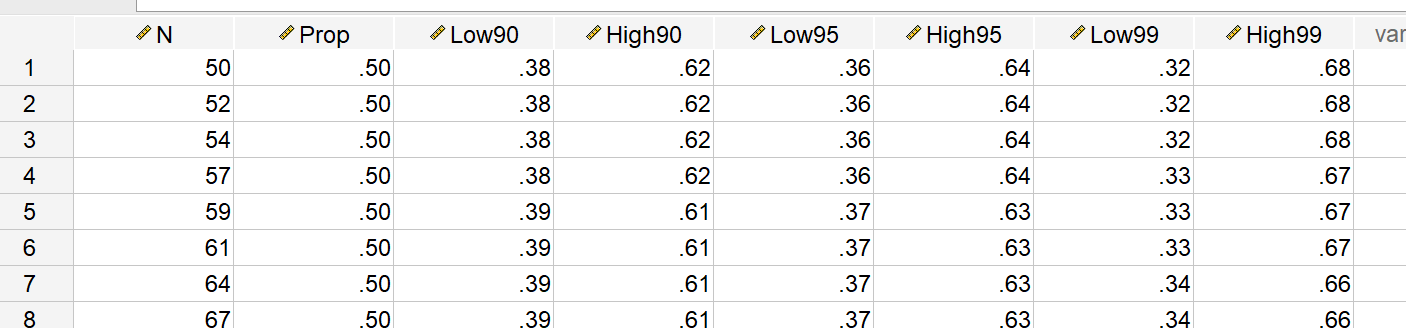For sample sizes of closer to 3000, you can then see how these confidence intervals decrease in width to changes in 4 to 5%.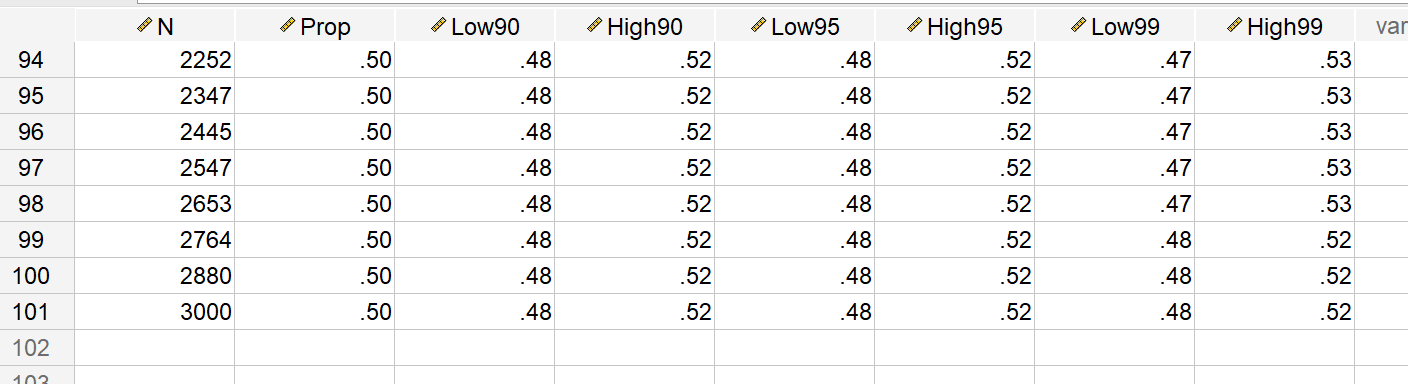But I really just generated the data this way to do a nice error bar chart to visualize:

``````*Precision chart.
GGRAPH
/GRAPHDATASET NAME="graphdataset" VARIABLES=Prop N Low90 High90 Low95 High95 Low99 High99
/GRAPHSPEC SOURCE=INLINE.
BEGIN GPL
SOURCE: s=userSource(id("graphdataset"))
DATA: N=col(source(s), name("N"))
DATA: Prop=col(source(s), name("Prop"))
DATA: Low90=col(source(s), name("Low90"))
DATA: High90=col(source(s), name("High90"))
DATA: Low95=col(source(s), name("Low95"))
DATA: High95=col(source(s), name("High95"))
DATA: Low99=col(source(s), name("Low99"))
DATA: High99=col(source(s), name("High99"))
DATA: N=col(source(s), name("N"))
GUIDE: text.title(label("Base Rate 50%"))
GUIDE: axis(dim(1), label("Sample Size"))
GUIDE: axis(dim(2), delta(0.05))
SCALE: linear(dim(1), min(50), max(3000))
transparency.interior(transparency."0.6"))
transparency.interior(transparency."0.6"))
transparency.interior(transparency."0.6"))
ELEMENT: line(position(N*Prop))
END GPL.``````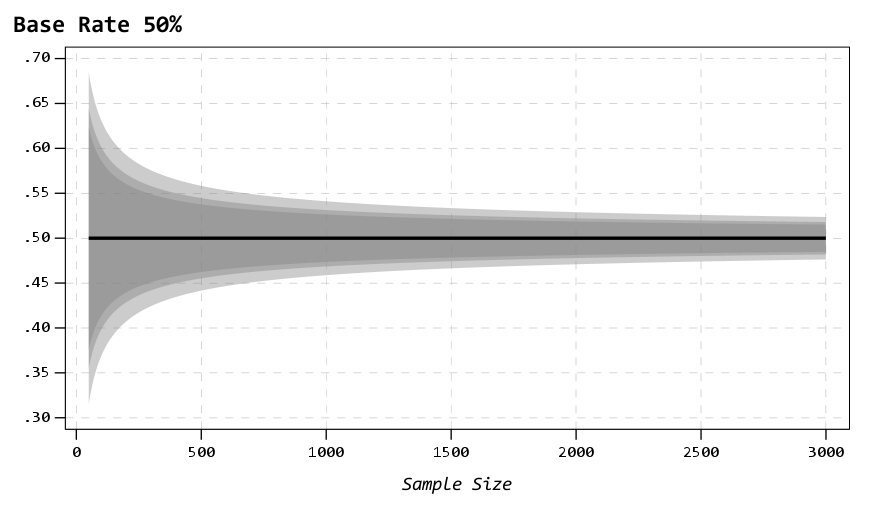So here the lightest gray area is the 99% CI, the second lightest is the 95%, and the darkest area is the 90% CI. So here you can make value trade offs, is it worth it to get an extra precision of under 10% by going from 500 samples to 1000 samples? Going from 1500 to 3000 you don’t gain as much precision as going from 500 to 1000 (diminishing returns).

It is easier to see the progression of the CI’s when plotting the sample size on a log scale (or sometimes square root), although often times costs of sampling are on the linear scale.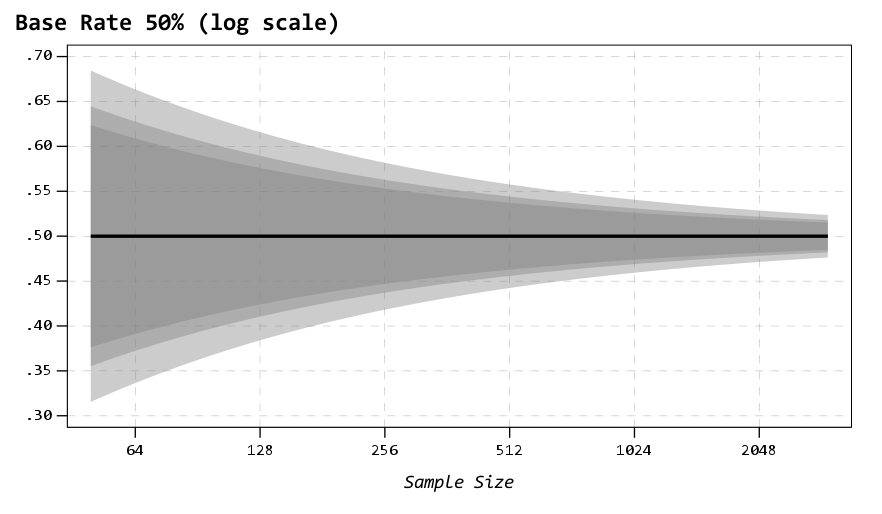You can then change the macro arguments if you know your baseline is likely to not be 50%, but say here 15%:

``!PrecisionProp Prop=0.15 MinN=50 MaxN=3000.``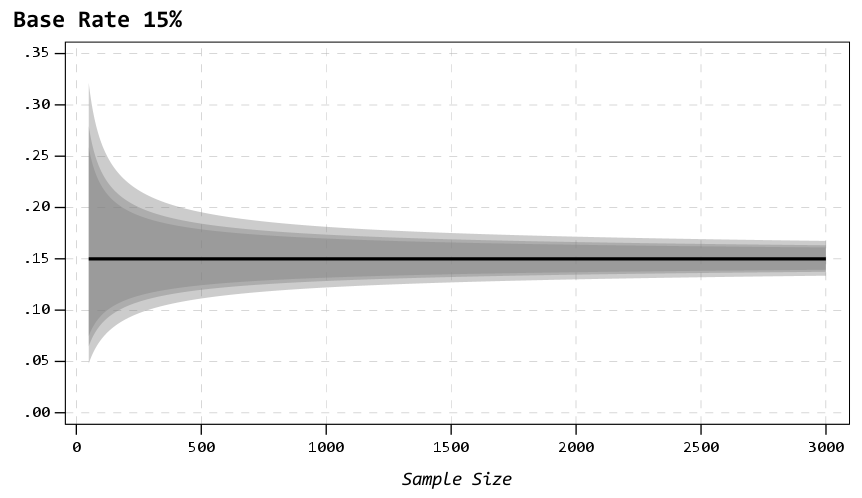So you can see in this graph that the confidence intervals are smaller in width than the 50% – a baseline of 50% results in the largest variance estimates for binary 0/1 data, so anything close to 0% or 100% will result in smaller confidence intervals. So this means if you know you want a precision of 5%, but only have a rough guess as to the overall proportion, planning for 50% baseline is the worst case scenario.

Also note that when going away from 50%, the confidence intervals are asymmetric. The interval above 15% is larger than the interval below.

A few times at work I have had pilots that look something like “historical hit rates for these audits are 10%, I want the new machine learning model to increase that to 15%”.

So here I can say, if we can only easily use a sample of 500 cases for the new machine learning model, the precision I expect to be around 11% to 19%. So cutting a bit close to be sure it is actually above the historical baseline rate of 10%. To get a more comfortable margin we need sample sizes of 1000+ in this scenario.

1.#### Paul Beaulne

/  November 10, 2021

This returns an error POWER PROPORTIONS INDEPENDENT /PARAMETERS TEST=NONDIRECTIONAL SIGNIFICANCE=0.05 POWER=0.6 0.7 0.8 0.9 NRATIO=1 PROPORTIONS=0.1 0.2 METHOD=CHISQ ESTIMATE=NORMAL CONTINUITY=TRUE POOLED=TRUE /PLOT TOTAL_N.

Warnings Subcommand PARAMETERS: POWER must specify a single numerical value. Execution of this command stops.

•